Courses

# Kinetic Theory Of Gases MCQ Level – 1 (part - 2)

## 10 Questions MCQ Test Topic wise Tests for IIT JAM Physics | Kinetic Theory Of Gases MCQ Level – 1 (part - 2)

Description
This mock test of Kinetic Theory Of Gases MCQ Level – 1 (part - 2) for IIT JAM helps you for every IIT JAM entrance exam. This contains 10 Multiple Choice Questions for IIT JAM Kinetic Theory Of Gases MCQ Level – 1 (part - 2) (mcq) to study with solutions a complete question bank. The solved questions answers in this Kinetic Theory Of Gases MCQ Level – 1 (part - 2) quiz give you a good mix of easy questions and tough questions. IIT JAM students definitely take this Kinetic Theory Of Gases MCQ Level – 1 (part - 2) exercise for a better result in the exam. You can find other Kinetic Theory Of Gases MCQ Level – 1 (part - 2) extra questions, long questions & short questions for IIT JAM on EduRev as well by searching above.
QUESTION: 1

### The pressure P, volume V and temperature T of a gas in the jar A and other gas in the jar B at Pressure 2P, volume V/4 and temperature 2T, then the ratio of number of molecules in jar A and B will be. Select one:

Solution:

V = nRT    (n = number of moles)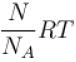(N = Number of molecules, NA = Avogadro number)
∴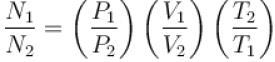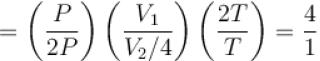The correct answer is: 4 : 1

QUESTION: 2

### The equation of state corresponding to 8gm of O2 is. Select one:

Solution:

As 32gm O2 means 1 mole of O2 molecule, therefore 8gm of O2 means 1/4 mole i.e., n = 1/4
∴ pV = nRT
⇒ pV = 1/4 RT
The correct answer is: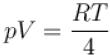QUESTION: 3

### The expansion of an ideal gas of mass m at a constant pressure P is given by the straight line D. Then the expansion of the same ideal gas of mass 2m at a pressure P/2 is given by the straight line.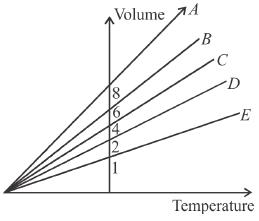Select one:

Solution:

From  PV α MT
or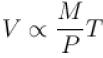Here (M/P) epresents the slope of curve drawn on volume and temperature axis.
For the first condition, slope (M/P) graph is D.  (given)
For second condition,
Slope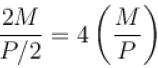i.e., slope becomes four times so graph A is correct
for this condition.
A

QUESTION: 4

At room temp. (300K), the rms speed of the molecules of certain diatomic gas is found to be 1930 m/s. The gas is
Select one:

Solution: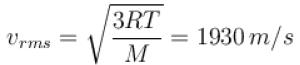⇒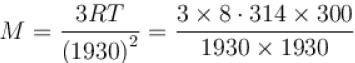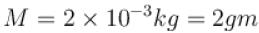⇒ gas is hydrogen.

QUESTION: 5

rms speed of gas molecule gas is v. If the pressure of the gas is doubled, what is the new rms speed?
Select one:

Solution: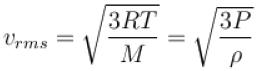rms speed of gas, molecules does not depend on the pressure of gas (if temperature remains constant) because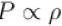(boyle’s law).
If pressure is increased n times then density will also increase by n times butrms speed remains constant.

QUESTION: 6

At top of a mountain, a thermometer reads 7°C and a barometer reads 70cm, of Hg. At the bottom of the mountain, these read 27°C and 76cm of Hg respectively.
Comparison of density of air at the top with that of bottom is.
Select one:

Solution:

Ideal gas equation, in terms of density is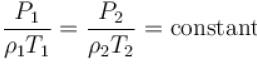⇒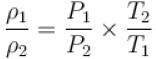⇒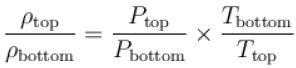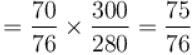QUESTION: 7

An experiment is carried on a fixed amount of gas at different temperature at high pressure such that it deviates from the ideal gas behavior. The variation of  with p is shown in the diagram. The correct variation will correspond to.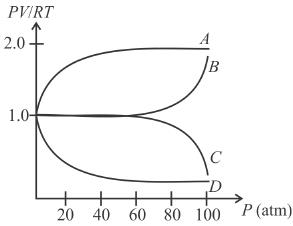Select one:

Solution:

At lower pressure, we can assume that given gas behaves as ideal gas so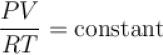but when pressure increase, the decrease in volume will not take place in same proportion so PV/RT will increase.
The correct answer is: Curve B

QUESTION: 8

Two containers of equal volume contain the same gas at pressure p1 and p2 and absolute temperature T1 and T2 respectively. On joining the vessels, the gas reaches a common pressure p and common temperature T. The ratio P/T is equal
to.
Select one:

Solution:

Number of moles in first vessel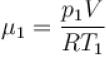and number of moles in 2nd  vessel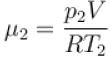If both vessels are joined together, then quantity of gas remains same i.e.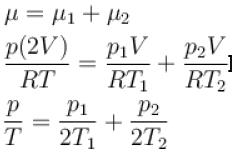The correct answer is: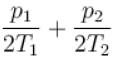QUESTION: 9

The temperature of a gas is raised while its volume remains constant, the pressure exerted by a gas on the walls of the container increases because its molecules.
Select one:

Solution:

Due to increase in temperature, root mean square velocity of gas molecule increases. So they strike the wall more often with higher velocity. Hence, the pressure exerted by a gas on the walls of the container increases.
The correct answer is: strike the wall more often with higher velocities

QUESTION: 10

The conversion of ideal gas into solids is.
Select one:

Solution:

Because there is zero attraction between the molecules of ideal gas.